#### Theory of DC Motors II, Torque and Speed

Introduction:

The aim of the motor is to exploit the interaction of magnetism and electricity to permit energy to be transformed from one form to another. Electrical energy is drawn by the motor and utilized to give mechanical energy as output. This mechanical energy is then utilized to do physical work of some sort. The electrical energy is drawn by armature winding of the motor that acts as the current carrying conductor positioned in the magnetic field. The stator field winding as well draws electrical energy when the magnets are electromagnets instead of permanent magnets. The mechanical energy is then provided as output through the torque produced by the rotating shaft as shown in figure below. The shaft can then be joined to a range of mechanical actuators to execute some task needing physical force or movement.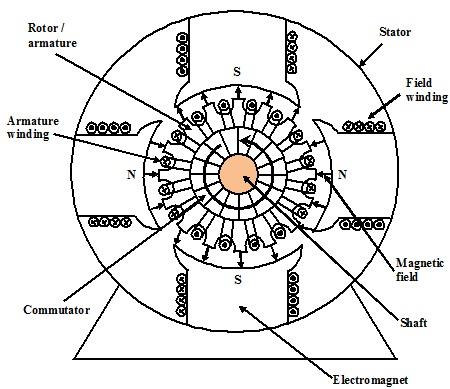Figure: An End-on View of Rotor and Stator of a DC Motor

Torque and Speed:

One form of electrical equivalent circuit for a motor is as shown in figure below. This is conventional and really shows the moving portions. Frequently, a coil is added to symbolize the field winding whenever the stator magnets are not permanent magnets however this is omitted here for clarity. The shaft of motor rotates at a rate stated by its angular velocity, ω. Though, the speed of rotation is very often provided in terms of revolutions per minute or rpm.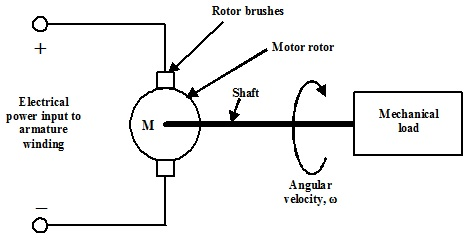Figure: Electrical Equivalent Representation of a Motor

From prior considerations of electromagnetism remember that the force generated on a conductor of length, l, carrying a current, I, in a magnetic field of flux density, B, has been provided as:

Force = Magnetic Flux density x Current x Length of Conductor x sin θ

F = B I l sin θ  N

Where Sin θ permits for angle between the direction of the magnetic field and the direction of travel of conductor. In the motor structure shown in figure above, the faces of the stator poles are curved and there are as well two sets of poles and hence even when the conductors in the armature rotate, their direction of travel is nearly constantly at right angles to the magnetic field. In this condition the factor Sin θ can be taken as unity and removed from the equation above. When there are N conductors mounted on an armature all carrying similar current, I, then the total force experienced by the combined armature winding is as follows:

F = B I N l  N

In case of a rotating machine, the mechanical load represents a moment of inertia to the circular movement of shaft and instead of a linear force; a rotational Torque is developed by the motor as shown in figure below. The torque is basically the turning or twisting of force experienced by a rotating body.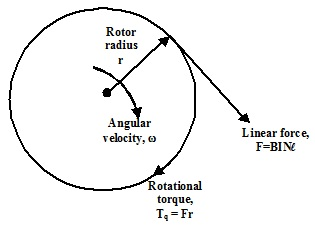Figure: Torque Developed by the rotation of rotor

The torque is associated to the force experienced by the conductors in an armature via its outer radius, r and hence:

Tq = Fr = B I N l r  Nm

The mechanical power delivered to the load is recognized by the torque developed in armature and its speed of rotation. This is illustrated as:

Power delivered to load = Torque developed x Angular velocity

PL = Tq ω  W

The angular velocity is usually termed to as the speed of motor. The speed of rotation expressed in revolutions per minute is represented as:

Speed of Rotation:  n rpm (revs per min.)

Then if, ω = 2π f, where f is the no. of cycles (or revolutions) per second and n is in revs per min this gives f = n / 60 and hence:

Angular velocity, ω = (2 Π n)/60 radians per second

Speed, n = (60ω)/(2 Π) revolutions per minute (rpm)

Output Power and Efficiency:

If the above relationship is replaced for angular velocity, then an expression for the output mechanical power accessible from the motor is as follows:

Mechanical power delivered to load PL = Tq ω = (2 Π n Tq)/60  W

This is the mechanical power delivered to load that can be thought of as the output power of motor. This power should be supplied as electrical power from dc supply employed to feed the windings of motor. In practice, not all the electrical power supplied to a motor is transformed into mechanical power accessible to the load. Some is lost as I2R losses in the copper wire of windings, some as leakage or eddy currents flowing in an armature and stator and a few due to friction. Thus more electrical energy is supplied as input to motor than is received as output mechanical energy. This leads to the idea of the Conversion Efficiency of a motor stated as:

Conversion Efficiency = (Mechanical output power delivered to load, PL)/ (Electrical input power drawn from supply, Pi) x 100%

Speed-Torque Characteristics:

If the motor consists of 100% conversion efficiency, the above power relationship recommends that for a given input electrical power, the output mechanical power must remain constant. Rewriting the relationship:

Torque, Tq = PL/ω  Nm

Therefore it can be seen that there is an inverse relationship between torque and speed for a fixed power input. This recommends that the torque must become infinite whenever the speed is decreased to zero, that is, beneath high-load conditions. On the contrary, if the torque is zero then the speed must become infinite, that is, beneath no-load conditions.

In practice, physical restrictions and finite efficiency prevent such extremes from being attained. In a real motor, there is still an inverse relationship which, however can be approximated by a straight line containing a negative slope. With speed as an independent variable:

Tq = m ω + C

Where m is negative and C is positive. This is plotted as shown in figure below.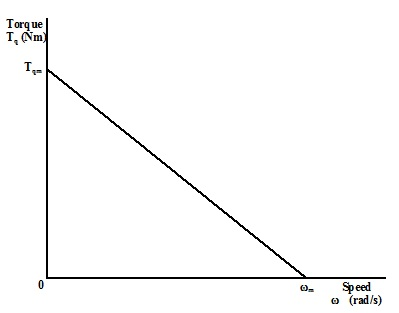Figure: The Speed-Torque Characteristic of a DC Motor

If ω = 0, Tq = C = Tqm

If Tq = 0, m ω + C = 0 and hence, ω = -(C/m) = - Tqm/m = ωm

And m = - Tqm/ ωm

This then provides the speed-torque relationship as follows:

Tq = (- Tqm/ ωm) ω + Tqm

The word ωm is the maximum rotational speed and is termed as the ‘no-load speed’.

The word Tqm is the maximum torque and is termed to as the ‘stall torque’.

This as well provides a revised expression for the power delivered to load as shown:

Power delivered to load, PL = Tq ω = (- Tqm/ ωm) ω2 + Tqm ω  W

To find out the conditions for maximum power output to the load, the derivative of this expression can be recognized and equated to zero as:

dPL/dω = - 2(Tqm/ ωm) ω + Tqm = 0

That gives,

2 (Tqmm) = Tqm

And hence, ω = (Tqm/Tqm) (ωm/2) = ωm/2

This points out that the motor must be operated at half of its maximum speed in order to receive the maximum mechanical power output.

Beneath this condition the maximum power received is found as:

Maximum power PL MAX = (- Tqm/ ωm) (ωm2/4) + Tqmm/2)   W

And hence,

Maximum power, PL MAX = (- Tqm ωm/4) + (Tqm ωm/2)   W

That gives,

Maximum power, PL MAX = (Tqm ωm/4) = (Tqm/2) x (ωm/2)  W

The power can be seen to be explained by the region of the rectangle made by the coordinates of the operating point. The above analysis exhibits that in order to receive maximum mechanical power output to the load; the motor must be operated at coordinates of half maximum speed and half maximum torque as shown in figure below. When the output power is plotted as the function of speed, this can be seen to be a parabolic function.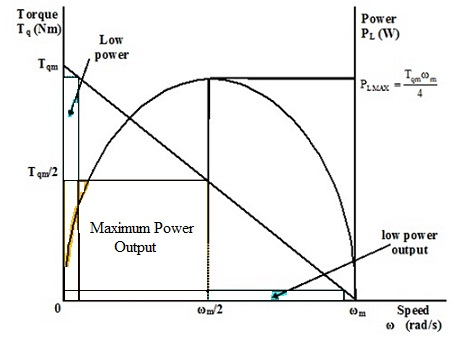Figure: Operation of a DC Motor for Maximum Power Output

Illustration:

The starter motor in a car should turn the engine throughout start-up at a speed of 400rpm. The engine is driven directly from dc motor that draws a power of 2.5kW from the battery. The motor consists of a stator consists of four permanent magnets that provide a total magnetic flux of 0.04Wb surrounding the rotor. The rotor consists of an armature of 120mm length and 6cm diameter that carries a winding comprising of 20 conducting elements.

Find out the torque developed by the motor and current needed in the armature winding if the conversion efficiency of the motor is 90 percent.

Solution:

Electrical power input to the motor is Pi = 2.5kW and η = 90% = 0.90

Mechanical power accessible to the engine is PL = 0.90 x 2500 = 2250W

The start-up speed is provided as:

ω = (2 Π n)/60 = (2 x 3.14 x 400)/60 = 41.9 rad/s

Torque developed by the motor is:

Tq = PL/ω = 2250/41.9 = 53.7  Nm

The relationship between torque and linear force provides:

Tq = Fr Nm that gives F = Tq/r = 53.7/0.03 = 1790 N

But, F = B I N L  N, where B = Φ/A and Φ = 0.04 Wb

The area, A, should be computed as half the surface area of the armature treated as a cylinder as there are two north poles and two south poles and hence the flux enters via half of the surface and exits via the other half of the surface. The cylinder is of length, h = 120mm and diameter, d = 6cm and hence:

A = 0.5πdh = 0.5 x 3.14 x 0.06 x 0.12 = 0.011 m2.

Then, B = Φ/A = 0.04/0.011 = 3.64 Wb/m2

And finally, F = B I N l, then I = F/(BNl) = 1790/(3.64 x 20 x 0.12) = 205 A

Latest technology based Electrical Engineering Online Tutoring Assistance

Tutors, at the www.tutorsglobe.com, take pledge to provide full satisfaction and assurance in Electrical Engineering help via online tutoring. Students are getting 100% satisfaction by online tutors across the globe. Here you can get homework help for Electrical Engineering, project ideas and tutorials. We provide email based Electrical Engineering help. You can join us to ask queries 24x7 with live, experienced and qualified online tutors specialized in Electrical Engineering. Through Online Tutoring, you would be able to complete your homework or assignments at your home. Tutors at the TutorsGlobe are committed to provide the best quality online tutoring assistance for Electrical Engineering Homework help and assignment help services. They use their experience, as they have solved thousands of the Electrical Engineering assignments, which may help you to solve your complex issues of Electrical Engineering. TutorsGlobe assure for the best quality compliance to your homework. Compromise with quality is not in our dictionary. If we feel that we are not able to provide the homework help as per the deadline or given instruction by the student, we refund the money of the student without any delay.